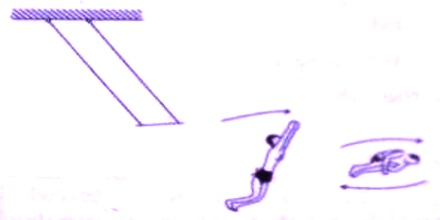# Conservation of Angular Momentum

Conservation of angular momentum

From Newton’s first law for angular motion we know that change of angular velocity i.e., angular momentum occurs due to the action of external torque only. If there is no torque, the body will rotate in uniform angular velocity. That means angular velocity with respect to time becomes constant. Hence, angular momentum also becomes constant. This is called conservation principle of angular momentum. So it can be said that if resultant of the torque on a body is zero, the angular momentum remains conserved.

Mathematical proof: We know angular momentum, L = Iω … … … (1)

Here L is the angular momentum of the body, ‘I’ is the moment of inertia and ω is the angular velocity. Differentiating equation (1) with respect to time,

dL/dt = d/dt (Iω) = I dω/dt

But, dω/dt = α

so, dL/dt = Iα = τ     (according to Newton’s second law of Angular motion)

Now, τ = 0, i.e., if torque is not active on the body.

then, dL/dt = 0; L = constant.

Hence, if the resultant torque acting on a body is zero, there will not be any change of angular momentum. This is the conservation principle of angular momentum.

Example: You have seen trafiz game in the circus. Their players demonstrate many exercises in air. While pumping from a swinging pad the hands and feet of the player remain stretched. At this time his angular velocity is minimum. Now when hands and feet are folded and brought near to the chest, the angular velocity increases; so, it becomes easier for him to somersault in air successively. Due to folding of hands and feet moment of inertia (I) of the player becomes less, but as his angular momentum L = lω remains constant so his angular velocity increases [Figure].